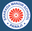Trending ▼   ResFinder# ISC Class XII Prelims 2017 : Mathematics (Sharada Mandir School ICSE, Miramar Panaji, Goa)

3 pages, 40 questions, 0 questions with responses, 0 total responses,20Joy Kapadia Sharada Mandir School ICSE, Miramar Panaji, Goa
+Fave Message
 Home > joykapadia >   F Also featured on: School Page iscFormatting page ...

Class 12 SHARADA MANDIR SCHOOL First Preliminary Examination November 2016 MATHEMATICS PAPER (3 HOURS) Max Marks -100 Section A Answer Question 1 (compulsory) and Five other questions. Section C Answer two questions from either Section C. All working, including rough work, should be done on the same sheet as, and adjacent to, the rest of the answer. The intended marks for questions or parts of questions are given in brackets [ ]. .. SECTION A Question 1. [10 3 = 30] i. Find the value of k if M = ii. Find the equation of tangent to the ellipse axis iii. Evaluate: iv. Solve v. Evaluate the integral vi. Evaluate vii. Find the regression coefficient byx and bxy ,if , , , and n=6. Also find the correlation coefficient r A machine manufactured by a firm consists of two parts A and B. But out of 100 A s manufactured 9 are likely to be defective and out of 100 B s manufactured, 5 are likely to be defective. Then find the probability that a machine manufactured by the firm is free from any defect viii. = ,then find the value of x which makes an angle of 30 with the x- ix. Express the complex number in the polar form x. Find the equation of hyperbola with centre at the origin, the length of transverse axis is 6 and one focus at (0,4) Question 2. (a) Show that = (a+b+c) (  (b) Using matrices solve the following system of equations: 3x-2y+z=2, 2x+y-3z = -5, -x+2y+z=6Formatting page ...Formatting page ...